Test: Differential Equations & Multiple Integrals- 1

# Test: Differential Equations & Multiple Integrals- 1

Test Description

## 20 Questions MCQ Test GATE Mechanical (ME) 2023 Mock Test Series | Test: Differential Equations & Multiple Integrals- 1

Test: Differential Equations & Multiple Integrals- 1 for Civil Engineering (CE) 2023 is part of GATE Mechanical (ME) 2023 Mock Test Series preparation. The Test: Differential Equations & Multiple Integrals- 1 questions and answers have been prepared according to the Civil Engineering (CE) exam syllabus.The Test: Differential Equations & Multiple Integrals- 1 MCQs are made for Civil Engineering (CE) 2023 Exam. Find important definitions, questions, notes, meanings, examples, exercises, MCQs and online tests for Test: Differential Equations & Multiple Integrals- 1 below.
Solutions of Test: Differential Equations & Multiple Integrals- 1 questions in English are available as part of our GATE Mechanical (ME) 2023 Mock Test Series for Civil Engineering (CE) & Test: Differential Equations & Multiple Integrals- 1 solutions in Hindi for GATE Mechanical (ME) 2023 Mock Test Series course. Download more important topics, notes, lectures and mock test series for Civil Engineering (CE) Exam by signing up for free. Attempt Test: Differential Equations & Multiple Integrals- 1 | 20 questions in 60 minutes | Mock test for Civil Engineering (CE) preparation | Free important questions MCQ to study GATE Mechanical (ME) 2023 Mock Test Series for Civil Engineering (CE) Exam | Download free PDF with solutions
 1 Crore+ students have signed up on EduRev. Have you?
Test: Differential Equations & Multiple Integrals- 1 - Question 1

### A triangle ABC consists of vertex points A (0,0) B(1,0) and C(0,1). The value of the integral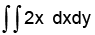over the triangle is

Detailed Solution for Test: Differential Equations & Multiple Integrals- 1 - Question 1

The equation of the line  AB is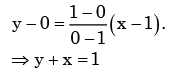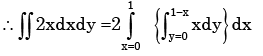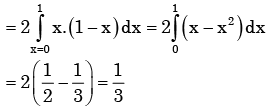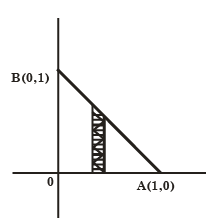Test: Differential Equations & Multiple Integrals- 1 - Question 2

### The area enclosed between the parabala y = x2 and the straight line     y = x is

Detailed Solution for Test: Differential Equations & Multiple Integrals- 1 - Question 2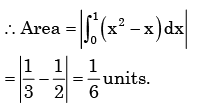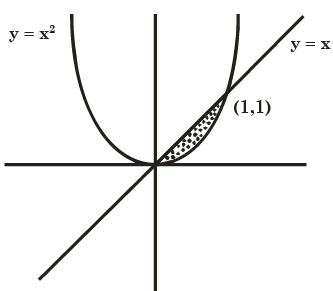Test: Differential Equations & Multiple Integrals- 1 - Question 3

### Changing the order of the integration in the double integral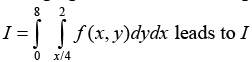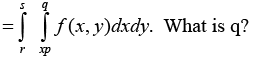Detailed Solution for Test: Differential Equations & Multiple Integrals- 1 - Question 3

When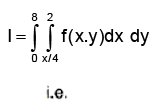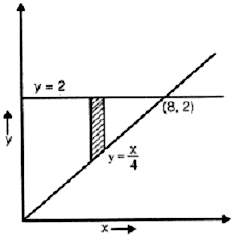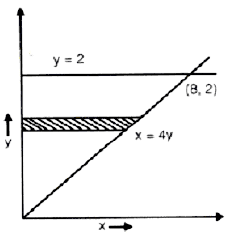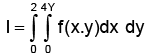Test: Differential Equations & Multiple Integrals- 1 - Question 4

The right circular cone of largest volume that can be enclosed by a sphere of 1 m radius has a height of

Detailed Solution for Test: Differential Equations & Multiple Integrals- 1 - Question 4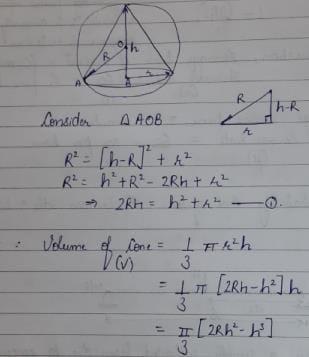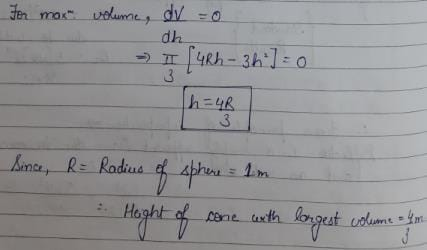Test: Differential Equations & Multiple Integrals- 1 - Question 5

the parabolic arc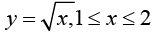is revolved around the x-axis. The volume of

Detailed Solution for Test: Differential Equations & Multiple Integrals- 1 - Question 5

Differential volume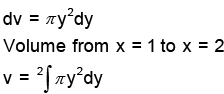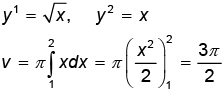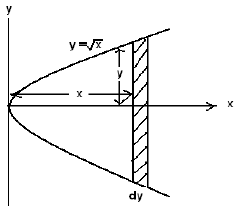Test: Differential Equations & Multiple Integrals- 1 - Question 6

What is the area common to the circles r = a and r = 2a cos θ?

Detailed Solution for Test: Differential Equations & Multiple Integrals- 1 - Question 6

Area common to circles r = a

And r = 2a cos θ is 1.228 a2

Test: Differential Equations & Multiple Integrals- 1 - Question 7

The expression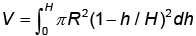for the volume of a cone is equal to

Detailed Solution for Test: Differential Equations & Multiple Integrals- 1 - Question 7

Choices (a) and (b)  be correct because

for the volume of a cone is equal to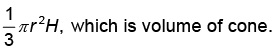Test: Differential Equations & Multiple Integrals- 1 - Question 8

f ( x,y ) is a continuous defined over ( x,y ) ∈ [0,1]× [0,1] . Given two constrains, x > y 2 and y > x 2 , the volume under f ( x,y ) is

Detailed Solution for Test: Differential Equations & Multiple Integrals- 1 - Question 8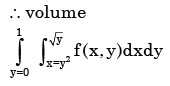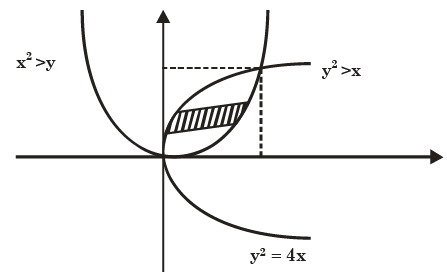Test: Differential Equations & Multiple Integrals- 1 - Question 9

The following differential equation has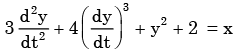Detailed Solution for Test: Differential Equations & Multiple Integrals- 1 - Question 9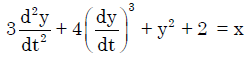Order of highest derivative = 2
Hence, most appropriate answer is (b)

Test: Differential Equations & Multiple Integrals- 1 - Question 10

A solution of the following differential equation is given by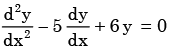Detailed Solution for Test: Differential Equations & Multiple Integrals- 1 - Question 10

Let y = mx be the trial soln of the given differental equation
∴The corrosponding auxiliary equation is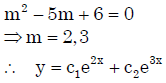Test: Differential Equations & Multiple Integrals- 1 - Question 11

For the differential equation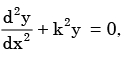the boundary conditions are

(i)  y = 0 for x = 0, and

(ii) y = 0 for x = a

The form of non-zero solutions of y (where m varies over all integers) are

Detailed Solution for Test: Differential Equations & Multiple Integrals- 1 - Question 11

Here y = c1 coskx + c2 sinkx ........... (1) be the solution of the given differential equation.
Now use boundary conditions
For x = 0,y = 0 gives c1 = 0. Equation − (1) becomes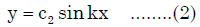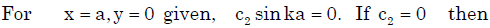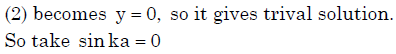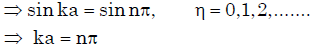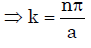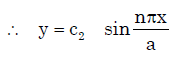be the solution, n 0,1,2,3.......

Test: Differential Equations & Multiple Integrals- 1 - Question 12

Which of the following is a solution to the differential equation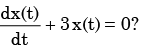Detailed Solution for Test: Differential Equations & Multiple Integrals- 1 - Question 12

Hints : m + 3 = 0 ⇒m = −3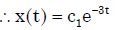Test: Differential Equations & Multiple Integrals- 1 - Question 13

For the differential equation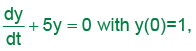the general solution is

Detailed Solution for Test: Differential Equations & Multiple Integrals- 1 - Question 13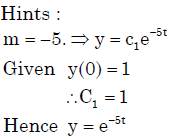Test: Differential Equations & Multiple Integrals- 1 - Question 14

The solution of the differential equation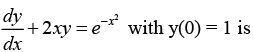Detailed Solution for Test: Differential Equations & Multiple Integrals- 1 - Question 14

It is a linear diff. equation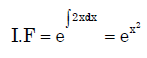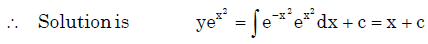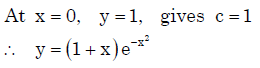Test: Differential Equations & Multiple Integrals- 1 - Question 15

The solution of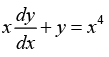with the condition y(1)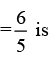Detailed Solution for Test: Differential Equations & Multiple Integrals- 1 - Question 15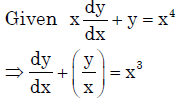Which is 1st order linear differential equation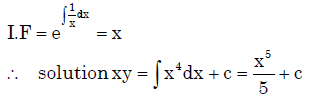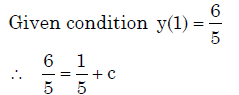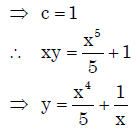Test: Differential Equations & Multiple Integrals- 1 - Question 16

A technique by which problems in analysis, in particular differential equations, are transformed into algebraic problems is called

Test: Differential Equations & Multiple Integrals- 1 - Question 17

The general solution of (x2 D2 – xD), y= 0 is :

Test: Differential Equations & Multiple Integrals- 1 - Question 18

For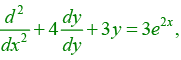the particular integrals is

Detailed Solution for Test: Differential Equations & Multiple Integrals- 1 - Question 18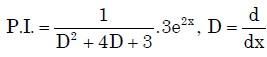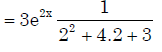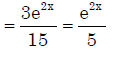Test: Differential Equations & Multiple Integrals- 1 - Question 19

It is given that y" + 2y' + y = 0, y(0) = 0, y(1)=0. What is y (0.5)?

Detailed Solution for Test: Differential Equations & Multiple Integrals- 1 - Question 19

Auxiliary equation is m2 + 2m +1 = 0⇒m = −1,−1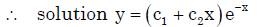Using boundary condition y(0) = 0 and y(1) = 0
we get y = 0

Test: Differential Equations & Multiple Integrals- 1 - Question 20

The partial differential equation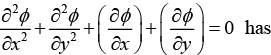Detailed Solution for Test: Differential Equations & Multiple Integrals- 1 - Question 20

The order is the highest numbered derivative in the equation (which is 2 here), while the degree is the highest power to which a derivative is raised (which is 1 here).

## GATE Mechanical (ME) 2023 Mock Test Series

27 docs|243 tests
 Use Code STAYHOME200 and get INR 200 additional OFF Use Coupon Code
Information about Test: Differential Equations & Multiple Integrals- 1 Page
In this test you can find the Exam questions for Test: Differential Equations & Multiple Integrals- 1 solved & explained in the simplest way possible. Besides giving Questions and answers for Test: Differential Equations & Multiple Integrals- 1, EduRev gives you an ample number of Online tests for practice

## GATE Mechanical (ME) 2023 Mock Test Series

27 docs|243 tests